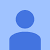# Why we use wildacar mask instead of subnet mask in ospf & eigrp roting protocols ..?• 4925,
CCNA Routing and Switching

As of use both wildcard mask and subnet masks are same but

- when we are calculating subnet mask  we make network bits = 1 and host bits =0  . ex. ip = 11.11.11.11 subnet mask = 255.255.255.240 here numbers of 1's = 28 so we can say number of network bits in ip address are 28 and rest 4 bits are hosts bit.

- Wildcard Mask can be calculated as network bits = 0 and host bits = 1 so we can say that  wildcard mask is just oppsite to subnet mask.

while some protocols like eigrp , ospf ,bgp use wildcard mask in their network command to enable these protocols on specific interfaces instead on complete router .

ex. ip = 11.11.11.11   its subnet mask = 255.255.255.240 so its wildcard mask will be = 0.0.0.15

how to calculate wildcard mask from subnet  mask in above example =  first ocet( 255-255 ) . second octet ( 255-255 ) . third octet ( 255-255 ) . fourth octet ( 255- 240 ).

now Routing Protocol uses wildcard mask :

example in eigrp :

network 192.168.1.0 0.0.0.3    will enable eigrp on interfaces having ip addr 192.168.1.1 and 192.168.1.2

Recommended